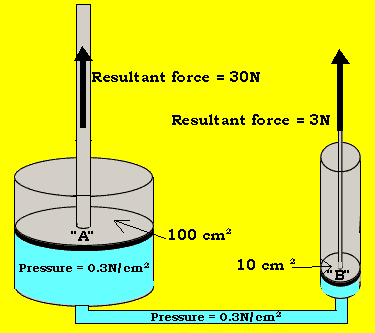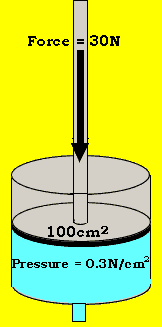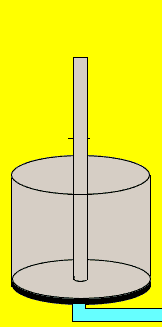Hydraulics Force, Work and Pressure. The force produced by a piston depends on the pressure the liquid inside the piston is under and the surface area of the piston. The force can be calculated according to the expression below. Force = pressure X area Look at the single pistons on the right. Piston "A" has a surface area of 100 cm2 and fluid at a pressure of 0.3N / cm2. The resultant force is calculated as follows Force = 0.3N/cm2 X 100cm2 Force = 30NPressure is the force exerted per unit area. Obviously the greater the force exerted over a small area the greater the pressure. The piston on the left has a surface are of 100 cm2and is pushed down with a force of 30 N. The pressure that the fluid inside the piston is under can be calculated by the expression below Pressure = force / area Pressure = 30N / 100 = 0.3N/cm2Consider carrying 25 Kg of bricks 25 metres along the road. You can say you have done some work. Now try carrying 25Kg of bricks 50 metres along the road. You can now say you have done more work than before. Work is a concept defined by the expression below. Work = force(N) X distance(m). So the work done on the bricks to carry them 25 metres along the road is the product of the force, exerted by the man to lift the bricks against gravtiy and the distance travelled. The unit for work is the joule. One joule of work is done when a force of one Newton is applied over a distance of one metre. Consider the piston on the left. If a force of 3N is applied to lift an object resting on the piston 3m high the work done on the object is Work = 3N X 3m = 9Nm = 9Joules. Continue with Hydraulics - transmitting forces through liquids.

Home